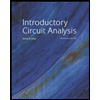# The ABCD constants of a three-phase, 345-kV transmission line are A-D-0.98 182 + 10.00 12447 B-4.035 + j58947 C j0.00061137 The line delivers 400 MVA at 0.8 lagging power factor at 345 kV. Determine the sending end quantities, voltage regulation, and transmission efficiency. Hint: Efficiency is equal to the ratio of active power at the receiving end to the active power at the sending end.

Question

The ABCD constants of a three-phase, 345-kV transmission line are:
A = D = 0.98182 + j0.0012447.
B = 4.035 + j58.947.
C = j0.00061137.
The line delivers 400 MVA at 0.8 lagging power factor at 345 kV. Determine the sending end quantities, voltage regulation, and transmission efficiency.
Hint: Efficiency is equal to the ratio of active power at the receiving end to the active power at the sending end.

Expert Solution

### Want to see the full answer?

Check out a sample Q&A hereStudents who’ve seen this question also like:Introductory Circuit Analysis (13th Edition)
13th Edition
ISBN: 9780133923605